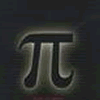#### You may also like### Approximations, Euclid's Algorithm & Continued Fractions

This article sets some puzzles and describes how Euclid's algorithm and continued fractions are related.### Euclid's Algorithm II

We continue the discussion given in Euclid's Algorithm I, and here we shall discover when an equation of the form ax+by=c has no solutions, and when it has infinitely many solutions.### Solving with Euclid's Algorithm

A java applet that takes you through the steps needed to solve a Diophantine equation of the form Px+Qy=1 using Euclid's algorithm.

# BT.. Eat Your Heart Out

##### Age 16 to 18 Challenge Level:

My phone number has seven digits: if the last four digits are placed in front of the remaining three you get one more than twice my number! What is the number?